# Chapter 5

## Chapter 5 Code Examples

### MATLAB Code Examples

x=[1 2 3 5 7 7.5 8 1];
y=[2 6.5 7 7 5.5 4 6 8];
plot(x,y)
year=[1988:1:1994];
sales=[127 130 136 145 158 178 211];
plot(year, sales, '--r*')
x=[-2: 0.01: 4];
y=3.5 .^ (-0.5*x) .* cos(6*x); plot(x,y)
x=[-2:0.01:4];
y=3*x.^3-26*x+6;
yd=9*x.^2-26;
ydd=18*x;
plot(x,y,'-b',x,yd,'--r',x,ydd,':k')
x=[10:0.1:22];
y=95000./x.^2;
x_data=[10:2:22];
y_data=[950 640 460 340 250 180 140];
plot(x,y,'-')
hold on
plot(x_data,y_data,'ro--')
hold off
xlabel('DISTANCE (cm)')
ylabel('INTENSITY (lux)')
title('Light Intensity as a Function of Distance')
axis([8 24 0 1200])
legend('Theory','Experiment')

### Python Code Examples

x=np.array([1,2,3,5,7,7.5,8,10])
y=np.array([2,6.5,7,7,5.5,4,6,8])
plt.plot(x,y)
year=np.arange(1988,1994.1,1)
sales=np.array([127,130,136,145,158,178,211])
plot(year, sales, '--r*')
x=np.arange(-2,4.001,.01)
y=3.5**(-0.5*x)*np.cos(6*x)
plt.plot(x,y)
x=np.arange(-2,4.001,.01)
y=3*x**3-26*x+6
yd=9*x**2-26
ydd=18*x
plt.plot(x,y,'-b',x,yd,'--r',x,ydd,':k')
x=np.arange(10,22.01,0.1)
y=95000/x**2;
x_data=np.arange(10,22.01,2)
y_data=np.array([950,640,460,340,250,180,140])
plt.plot(x,y,'-',label='Theory')
plt.plot(x_data,y_data,'ro--',label='Experiment')
plt.xlabel('DISTANCE (cm)')
plt.ylabel('INTENSITY (lux)')
plt.title('Light Intensity as a Function of Distance')
plt.axis([8,24,0,1200])
plt.legend()

## Exercises

1. Make two separate plots of the function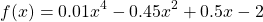one forxand one for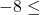x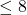2. Plot the function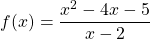Notice that the function has a vertical asymptote at x=2. Plot the function by creating two vectors for the domain of x, the first vector with elements from -4 to 1.7, and the second vector with elements from 2.3 to 8. For each x vector, create a y vector with the corresponding values of y according to the function. To plot the function make two curves in the same plot.
3. You have an electrical circuit that includes a voltage source vs with an internal resistance rs and a load resistance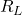. The power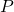dissipated in the load is given by: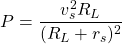Plot the poweras a function offor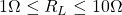given that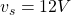and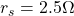4. The Gateway Arch in St. Louis is shaped according to the equation: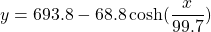Make a plot of the arch. Draw a horizontal line at ground level (y=0),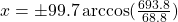5. In astronomy, the relationship between the relative temperature(temperature relative to the sun), relative luminosity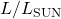and relative radius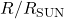of a star is modeled by: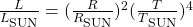The HR (Hertzsprung-Russell) diagram is a plot ofversus the temperature. The following data are given:

To compare the data with the model, plot a HR diagram. The diagram should have two sets of points. One uses the values offrom the table (use asterisk markers), and the other uses values ofthat are calculated by the equation usingfrom the table (use circle markers). In the HR diagram both axes are logarithmic. In addition, the values of the temperature on the horizontal axis are decreasing from left to right. Label the axes and use a legend. (See https://en.wikipedia.org/wiki/Hertzsprung%E2%80%93Russell_diagram for a general explanation of the HR diagram.)

1. The position x as a function of time that a particle moves along a straight line is given by: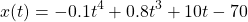The velocity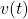is determined by the derivative of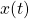with respect to, and the acceleration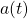is determined by the derivative ofwith respect to.
Derive the expressions for the velocity and acceleration of the particle, and make plots for the position, velocity, and acceleration as a function of time for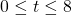. Timeis measured in seconds, and position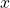is measured in meters. Make three plots, one for position, one for velocity, and one for acceleration. Label the axes appropriately with correct units.
2. A resistor,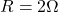and an inductor,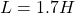are connected to a voltage source in series (RL circuit). When the voltage source applies a rectangular voltage pulse with an amplitude of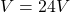and a duration of 0.5s, the current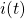in the circuit as a function of time is given by: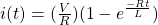for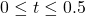for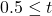Make a plot of the current as a function of time for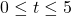seconds.